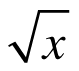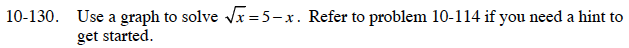### Home > CCA > Chapter 10 > Lesson 10.3.2 > Problem10-130

10-130.

Use a graph to solve= 5 − x. Refer to problem 10-114 if you need a hint to get started. 10-130 HW eTool (Desmos). Homework Help ✎$\text{Graph }y=5-x\text{ and }y=\sqrt{x}\text{ on the same set axes.}$

Where do the curves intersect?
If you were trying to solve the given equation, would you be solving for x or y?

x ≈ 3.2

Use the graphs below on the eTool help you with this problem.
Click on the link at right for the full eTool version: CCA 10-130 HW eTool